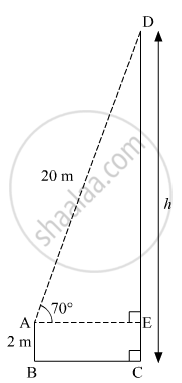Advertisement Remove all ads

# A Ladder on the Platform of a Fire Brigade Van Can Be Elevated at an Angle of 70° to the Maximum. the Length of the Ladder Can Be Extended Upto 20 M. If the Platform is 2m Above the Ground - Geometry

Advertisement Remove all ads
Advertisement Remove all ads
Advertisement Remove all ads

A ladder on the platform of a fire brigade van can be elevated at an angle of 70° to the maximum. The length of the ladder can be extended upto 20 m. If the platform is 2m above the ground, find the maximum height from the ground upto which the ladder can reach. (sin 70° = 0.94)

Advertisement Remove all ads

#### Solution

Let AB be the platform of the fire brigade van and AD be the ladder.
Suppose the maximum height from the ground upto which the ladder can reach be h m.Here, AB = 2 m, AD = 20 m, ∠DAE = 70º and AE ⊥ CD.
DE = CD − CE = (h − 2) m             (CE = AB)
In right ∆AED,
$\sin70^\circ = \frac{DE}{AD}$
$\Rightarrow 0 . 94 = \frac{h - 2}{20}$
$\Rightarrow h - 2 = 20 \times 0 . 94 = 18 . 8$
$\Rightarrow h = 18 . 8 + 2 = 20 . 8 m$

Thus, the maximum height from the ground upto which the ladder can reach is 20.8 m.

Concept: Heights and Distances
Is there an error in this question or solution?

#### APPEARS IN

Balbharati Mathematics 2 Geometry 10th Standard SSC Maharashtra State Board
Chapter 6 Trigonometry
Problem Set 6 | Q 9 | Page 139
Advertisement Remove all ads
Share
Notifications

View all notifications

Forgot password?